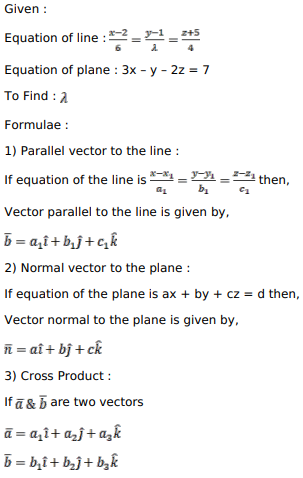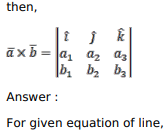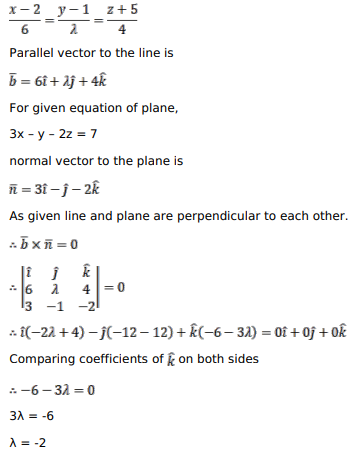# Solve this following

Question:

Find the value of $\lambda$ such that the line $\frac{x-2}{6}=\frac{y-1}{2}=\frac{z+5}{4}$ is perpendicular to the plane $3 x-y-2 z=7$.

Solution: